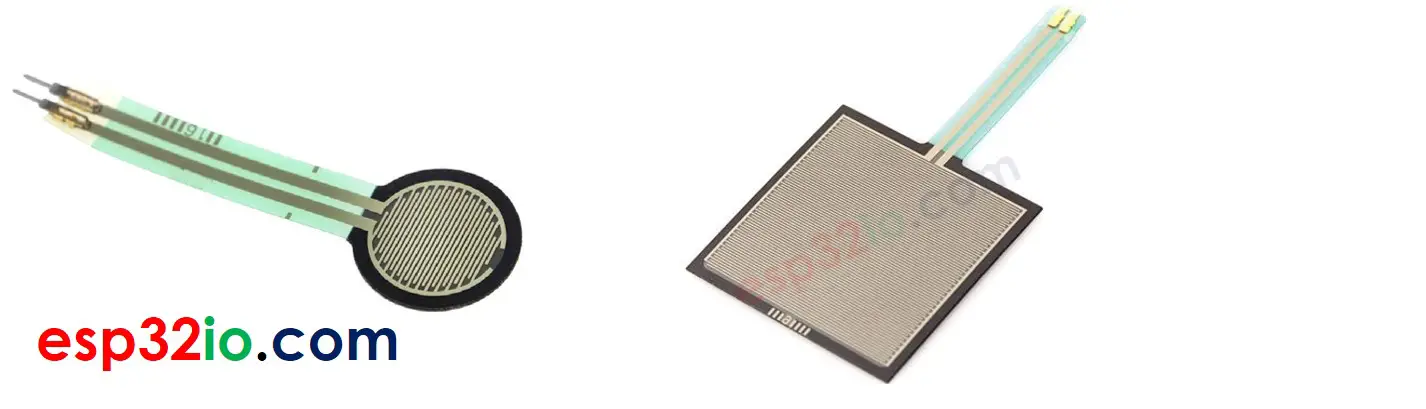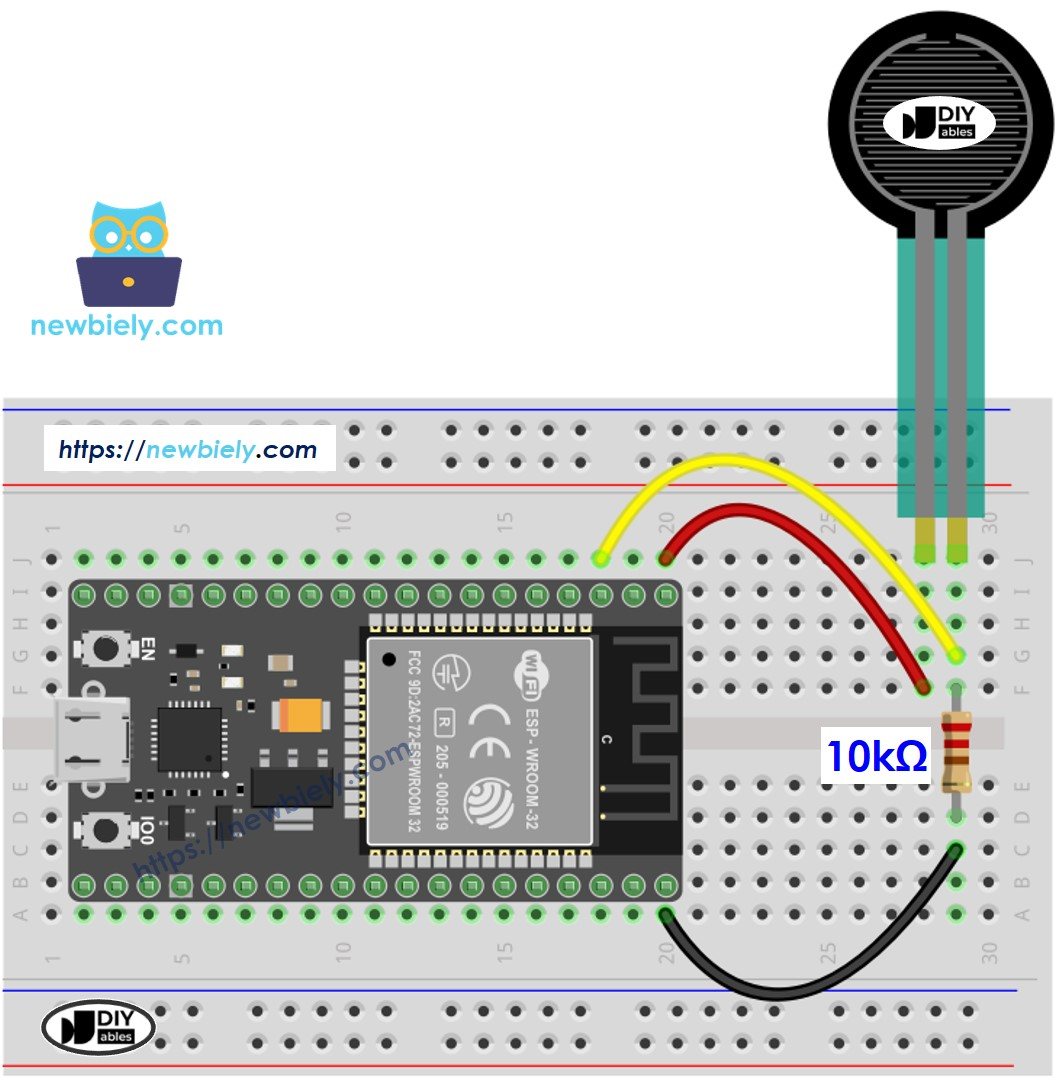# ESP32 - Force Sensor

The force sensor is called force sensitive resistor, the force sensing resistor, or FSR. This tutorial instructs you how to use ESP32 with the force sensor. In detail, we will learn:

• How the force sensor works.
• How to connect the force sensor to ESP32.
• How to program for ESP32 to read value from a force sensor.

## Hardware Used In This Tutorial

Or you can buy the following sensor kit:

Disclosure: some of these links are affiliate links. We may earn a commission on your purchase at no extra cost to you. We appreciate it.

## Introduction to Force Sensor

A force sensor is a resistor that its resistance is in inverse proportion to how much force it is given. The more force gives to the sensor, the smaller the sensor's resistance is. The force sensor is good for application that detects physical squeeze, pressure. However, it is not good for the application that finds how many pounds of weight

### Force Sensor Pinout

A force sensor has two pins. Just like a resistor, we do NOT need to differentiate these pins.## Wiring Diagram between Force Sensor and ESP32This image is created using Fritzing. Click to enlarge image

If you're unfamiliar with how to supply power to the ESP32 and other components, you can find guidance in the following tutorial: How to Power ESP32.

## How To Program Force Sensor

The resistance is in proportion to voltage. We can use the ESP32's analog input pin to measure voltage.

By connecting a pin of the force sensor to an analog input pin, we can read the analog value from the pin ⇒ voltage ⇒ resistance ⇒ force (all í in relative value)

## ESP32 Code

/* * This ESP32 code is created by esp32io.com * * This ESP32 code is released in the public domain * * For more detail (instruction and wiring diagram), visit https://esp32io.com/tutorials/esp32-force-sensor */ #define FORCE_SENSOR_PIN 36 // ESP32 pin GPIO36 (ADC0): the FSR and 10K pulldown are connected to A0 void setup() { Serial.begin(9600); } void loop() { int analogReading = analogRead(FORCE_SENSOR_PIN); Serial.print("The force sensor value = "); Serial.print(analogReading); // print the raw analog reading if (analogReading < 10) // from 0 to 9 Serial.println(" -> no pressure"); else if (analogReading < 200) // from 10 to 199 Serial.println(" -> light touch"); else if (analogReading < 500) // from 200 to 499 Serial.println(" -> light squeeze"); else if (analogReading < 800) // from 500 to 799 Serial.println(" -> medium squeeze"); else // from 800 to 1023 Serial.println(" -> big squeeze"); delay(1000); }

#### Quick Instructions

• If this is the first time you use ESP32, see how to setup environment for ESP32 on Arduino IDE.
• Copy the above code and paste it to Arduino IDE.
• Compile and upload code to ESP32 board by clicking Upload button on Arduino IDE
• Press the force sensor
• See the result on Serial Monitor. It looks like the below:.
COM6
The force sensor value = 0 -> no pressure The force sensor value = 0 -> no pressure The force sensor value = 132 -> light touch The force sensor value = 147 -> light touch The force sensor value = 394 -> light squeeze The force sensor value = 421 -> light squeeze The force sensor value = 607 -> medium squeeze The force sensor value = 791 -> medium squeeze The force sensor value = 921 -> big squeeze The force sensor value = 987 -> big squeeze The force sensor value = 0 -> no pressure The force sensor value = 0 -> no pressure
Autoscroll Show timestamp
Clear output
9600 baud
Newline

## Video Tutorial

Making video is a time-consuming work. If the video tutorial is necessary for your learning, please let us know by subscribing to our YouTube channel , If the demand for video is high, we will make the video tutorial.